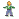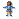## Family Magic

Follow the instruction below and I will tell you how many brothersand sistersyou have.
CLICK here to use your calculator

1. How many brothers do you have?  Ok good you know that.  Now add that number with 2.  That is X number of brothers (including you if you're a guy) plus 2.

2. Now multiply by 2 the number you got in 1.

3. Now add 1 to the number you got in 2.

4. Now multiply by 5 the number you got in 3 .

5. Now add the number of sisters (including you if you're a girl) to result in 4.

6. Now add 11 to the total you got in 5.

7. Ok are you sure you did the calculation correctly?  If so then enter it in the box below and click submit.

8. Alternative way:   where  #bro (including you if you are guy)
#sis (including you if you are girl)
parents = 2
(always)

9. TOTAL = ( ( ( ( (#bro + parents) * 2 ) + 1) * 5 ) + #sis) +11

1. An example of a family 6 with 2 sons and 2 daughters would be calculated as follows:

2. 2 sons plus 2 = 4.

3. 4 from (2) times 2=8.

4. 8 from (3) plus 1 =9.

5. 9 from (4) times 5 = 45.

6. 45 from (5) plus 2(girls) = 47.

7. 47 from (6) plus 11 = 58.

8. TOTAL = (  (  (  (  (2 + 2) * 2 ) + 1) * 5 ) + 2) +11 = 58

9. Now 58 is the number to be inserted in the box.

Note:  If your family members are more than 12 the magic wont work correctly.  It is only for normal families. ;P